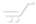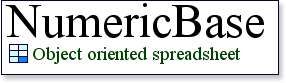# The NumericBase language

language basics
NumericBase saves solutions using a human readable text format. This means that you can either create solution using the user interface of NumericBase, or construct them yourself using a text editor. You can also write programs in other languages that dynamically generate NumericBase solutions, just like a web site script dynamically generate web page using the text-based HTML format.

This text format follows a specific syntax, called the NumericBase language. It includes table definitions, which contain information about tables in the solution, and display definitions, which contain information about the graphical layout of the solution. Any text that conform to this syntax is called a solution definition.

Names are used throughout the solution definition to name the various elements of the solution and to refer to them later. To use a name in a solution definition, you need to enclose it with brackets. If the name is a simple name, you can omit the brackets to make the solution definition more clear. This is the exact rule that apply to names that are used in formulas.

Formulas with syntax errors
As was mentioned in the "Formulas with errors" page , a NumericBase solution may contain formulas with syntax errors. To achieve this, NumericBase enclose these formulas with the sharp signs (#) to isolate them from the rest of the solution definition. In this way, NumericBase can load, or compile, a solution definition without getting confused by the syntax error of the formula.

For example: # 45+5+)#

If the formula does not have a syntax error, you can either enclose it with the sharp signs, or not. It does not change the meaning of the solution definition.

Table definition
The table definition contains information about one table in the solution. This information includes the table name, the base name, the names of the different columns, and all the formulas of the table. It has the several elements that must appear in the following order:
1. The "table" keyword.
2. The name of the table.
3. Optional extends clause that has the following elements:
• The "extend" keyword.
• The base name of the table.
4. The left brace sign {
5. (Optional) Any number of column definitions or row definitions. These definitions contains information about a specific column or row in the table and are described below.
6. The right brace sign }

For example:
table table1{ } table table2 extends table1{ }
Listing: table definitions

Column definition
The column definition contains information about one column in the table. More specifically, it has the column name, the formula at the base row of the column, and the "is visible" attribute of the column (determines whether or not the column is visible in a table view). Note that the column definition does not have the column number because NumericBase assigns column numbers according to the order in which the column definitions appear in the solution definition.

The syntax of column definition includes 4 elements that appear in the following order:
1. (Optional) column name followed by assignment operator (:=)
2. (Optional) The formula at the base row of the column.
3. If the column is visible then the exclamation point (!), otherwise, the semicolon sign (;).
Some examples are in the following table:

 column definition Remark simple_name:=43! Standard definition. 43! Column name is not present column_name :=! Column name is present but it does not have a formula at the base row. ! Column have neither name nor formula (perfectly valid) [column name]:=34+3! Column name is not a simple name so it is enclosed with brackets invisible_column := 34/column; Column is not visible because the definition ends with semicolon rather than exclamation point a:=#er+er)#! The formula at the base row of the column has a syntax error, so it is enclosed with the sharp signs.
Table: Examples of valid column definitions.

Another example is table definition with some column definitions, adopted from the circular dependency page:

table Table1{  //declaring the table (part of the table definition)   Celsius:=(Fahrenheit-32)*5/9 alt Kelvin-273!  //a column definition   Fahrenheit:=(Celsius*9/5)+32!  //another column definition   Kelvin:=Celsius+273!  //yet another column definition }  //the closing brace of the table definition
Listing: a table definition.

Row definition.
The row definition contain information about one row in the table. Each row has a corresponding row definition except for the base row. This is because the formulas of this row are represented by the column definition above.

Row definitions have the following syntax:
1. The less than sign: <
2. The row number (in case of base row, use sum instead)
3. The bigger than sign: >
4. The assignment operator :=
5. The left brace sign: {
6. (Optional) Any number of cell definitions. These definitions contain information about a specific cell and are described below.
7. The right brace sign: }

For example :
A row definition without any cell definitions:
<4> := {}

Cell definition
A cell definition appear in a row element only if the corresponding cell has a formula. It consist of two parts that appear in the following order:
1. Optional "column locator" that indicates the column of the cell. If not present, then the column is determined by the location of the cell definition within the row definition.
2. The formula of the cell.

The column locator can refer to the column either by name or by number. The two alternative are listed below:
1. By column name. consist of the followings parts:
• The column name.
• The assignment operator :=
2. By column number. consist of the followings parts:
• The less than sign: <
• The column number
• The bigger than sign: >
• The assignment operator :=
For example:

 column locator Remark <4>:=45+5 by column number [first name]:="ofer" by column name
Table: Examples of valid column locator

Examples for table definitions
The following listing shows a table definition that is based on the example shown at the end of circular dependency page. This listing adds a few row definitions to the listing above.

table Table1{    Celsius:=(Fahrenheit-32)*5/9 alt Kelvin-273!    Fahrenheit:=(Celsius*9/5)+32!    Kelvin:=Celsius+273!    <1>:={Celsius:=10,}   //a row definition    <2>:={Fahrenheit:=10,}   //a row definition    <3>:={Kelvin:=10,}   //a row definition }
Listing: a table definition.

display definitions
Display definitions control the graphical layout of the solution. More documentation on this subject will be ready soon.